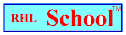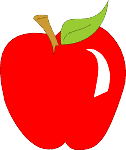Name_______________________________________________Date_________________________
Mathematics Problem Solving
Volume 5, Number 7, October 18, 1999
www.rhlschool.com

### How Can You Solve It?

1. Pamela has grown a 1,214 pound pumpkin. She’s very proud of it because the heaviest pumpkin she ever grew before weighed 34 pounds. She wants to tell her friends how many 34 pound pumpkins it would take to weigh as much as her new record setter. What is the best way to figure that out?

a. She should pile pumpkins that weigh 34 pounds on her bathroom scale until they weigh at least 1,214 pounds altogether.
b. She should subtract 34 from 1,214.
c. She should multiply 1,214 by 34.
d. She should divide 1,214 by 34.
e. She does not have enough information to figure it out.

2. Cheryl is going to bake 180 cupcakes for her Halloween party. Her recipe, for a dozen cupcakes, calls for two eggs. Which of the following methods should she use to figure out how many eggs she’ll need to make the 180 cupcakes?

a. She should just use 2 eggs and then observe how badly the cupcakes turn out.
b. She should divide 180 by 2.
c. She should multiply 180 by 2.
d. She should divide 180 by 12 and then multiply that number by 2.
e. She should multiply 180 by 12 and then add 2 to that number.

3. Robert has just finished picking a basket of apples. He knows that he has exactly 51 apples. The apples will cost 50 cents a pound. Robert wants toknow exactly how much the apples will cost before he pays the orchard owner. Using a calculator or just pencil and paper, what can you do to figure out the exact cost?

a. You can multiply \$ .50 by 51.
b. You can divide 51 by 16 and then multiply that number by \$ .50.
c. You can tell Robert to eat one apple so that the answer can come out evern.
d. You can multiply \$ .50 by the product of 51 and 16.
e. You don’t have enough information to figure out the answer.

4. Ryan wants to buy a gallon of cider. The cider costs severn dollars in a gallon container and \$ 2.27 in a quart container. Ryan knows that four quarts equal one gallon and four quart containers would fit better in his refrigerator. However, he wants to know how much money he can save by purchasing the gallon container instead. How can he figure that out?

a. He can multiply 4 by \$ 2.27 and then subtract \$ 7.00 from that amount.
b. He can add \$ 2.27 and \$ 7.00 and then divide that amount by 4.
c. He can buy five quarts and then subtract \$ 7.00 from whateverr they cost.
d. He does not have enough information to figure it out.
e. He can multiply \$ 7.00 by 4 and then divide by \$ 2.27.

rhlschool.com

RHL School - Free Worksheets and MoreCopyright 1999 RHL

RHL School Home